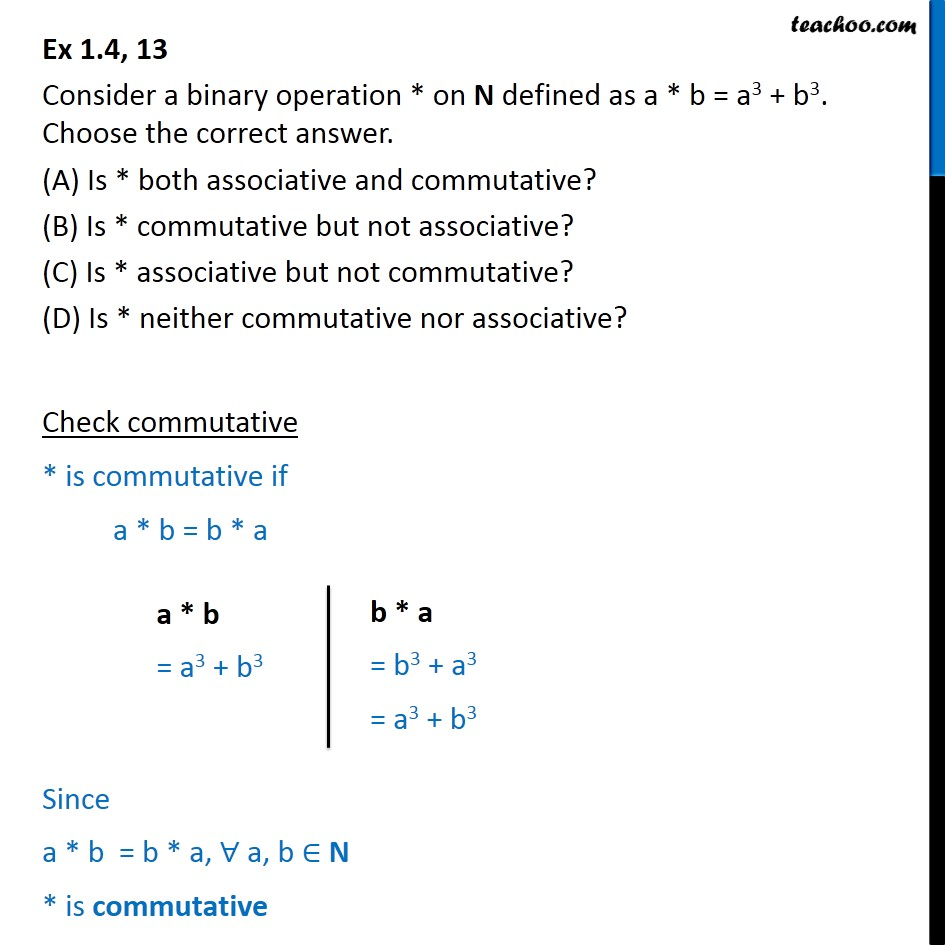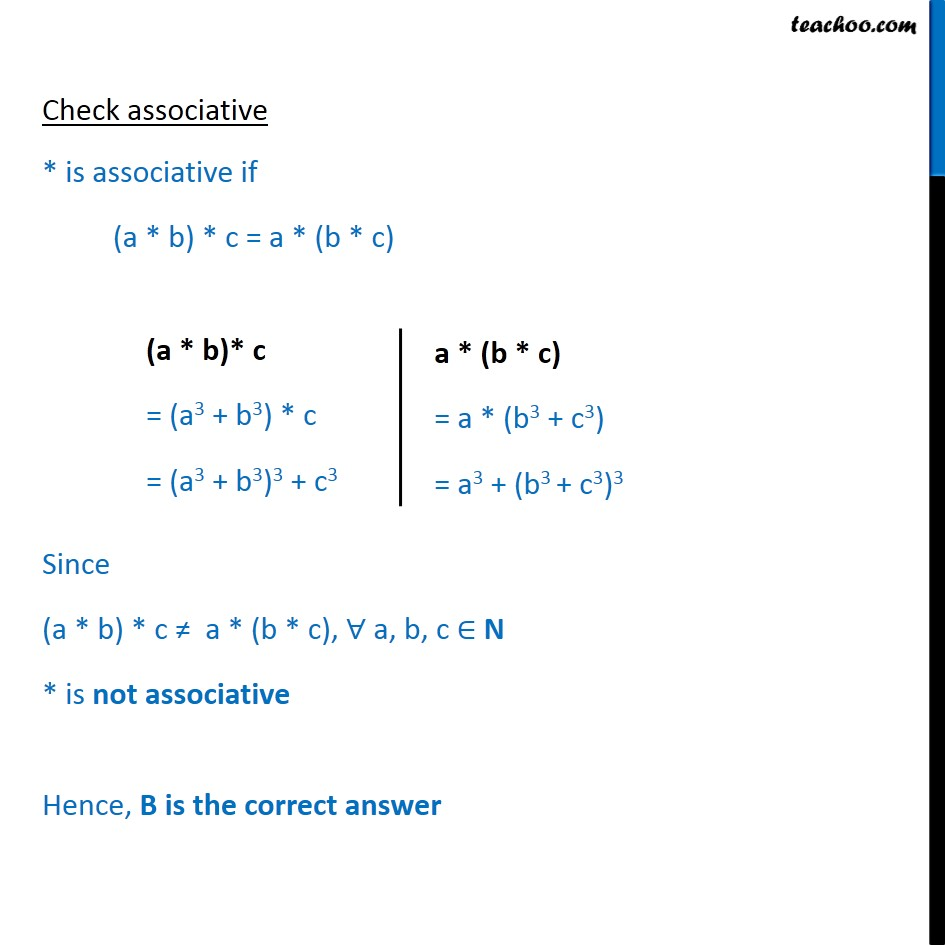Whether binary commutative/associative or not

Chapter 1 Class 12 Relation and Functions
Concept wiseIntroducing your new favourite teacher - Teachoo Black, at only ₹83 per month

### Transcript

Ex 1.4, 13 Consider a binary operation * on N defined as a * b = a3 + b3. Choose the correct answer. (A) Is * both associative and commutative? (B) Is * commutative but not associative? (C) Is * associative but not commutative? (D) Is * neither commutative nor associative? Check commutative * is commutative if a * b = b * a Since a * b = b * a, ∀ a, b ∈ N * is commutative Check associative * is associative if (a * b) * c = a * (b * c) Since (a * b) * c ≠ a * (b * c), ∀ a, b, c ∈ N * is not associative Hence, B is the correct answer